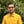Trusted answers to developer questions
Trusted Answers to Developer Questions

Related Tags

d
math
communitycreator

# What is hypot() in D?Harris Amjad

Grokking Modern System Design Interview for Engineers & Managers

Ace your System Design Interview and take your career to the next level. Learn to handle the design of applications like Netflix, Quora, Facebook, Uber, and many more in a 45-min interview. Learn the RESHADED framework for architecting web-scale applications by determining requirements, constraints, and assumptions before diving into a step-by-step design process.

### Overview

The longest side of a right-angled triangle, and the side opposite to the right angle, is known as the hypotenuse in geometry.

The mathematical formula to calculate the hypotenuse’s length is known as the Pythagorean theorem and is as follows:

hypotenuse = sqrt( length^2 + base^2 )


Figure 1 shows the visual representation of the hypot() function:

Figure 1: Visual representation of hypot() function

Note: We need to import std.math in our code to use the hypot() function. We can import it like this:
import std.math

### Syntax

hypot(length, base)


### Parameters

This function requires length and base as parameters.

### Return value

This function returns the hypotenuse of the right-angled triangle. The triangle’s length and base are passed as parameters.

### Example

The code below shows the use of the hypot() function in D:

import core.stdc.stdio;import std.stdio;//header required for functionimport std.math;int main(){    //positive: length     positive: base    writeln ("The value of hypot(10,10) : ",hypot(10,10));    //positive: length     negative: base    writeln ("The value of hypot(0.25,-2) : ",hypot(0.25,-2));    //negative: length     positive: base    writeln ("The value of hypot(-10,2) : ",hypot(-10,2));    //negative: length     negative: base    writeln ("The value of hypot(-0.5,-1) : ",hypot(-0.5,-1));    // few exceptional outputs    writeln ("The value of hypot(real.infinity,real.infinity) : ",hypot(real.infinity,real.infinity));    writeln ("The value of hypot(-real.infinity,-real.infinity) : ",hypot(-real.infinity,-real.infinity));    writeln ("The value of hypot(real.nan,real.nan) : ",hypot(real.nan,real.nan));    writeln ("The value of hypot(-real.nan,-real.nan) : ",hypot(-real.nan,-real.nan));    return 0;}
Get hypotenuse using hypot() function

### Explanation

• Line 4: We add the std.math library.
• Line 9: We calculate the hypotenuse of the positive length and the positive base using hypot().
• Line 12: We calculate the hypotenuse of the positive length and the negative base.
• Line 15: We calculate the hypotenuse of the negative length and the positive base.
• Line 18: We calculate the hypotenuse of the negative length and the negative base.
• Line 21 and onwards: We calculate the hypotenuse of exceptional numbers.

RELATED TAGS

d
math
communitycreator

CONTRIBUTORHarris Amjad

Grokking Modern System Design Interview for Engineers & Managers

Ace your System Design Interview and take your career to the next level. Learn to handle the design of applications like Netflix, Quora, Facebook, Uber, and many more in a 45-min interview. Learn the RESHADED framework for architecting web-scale applications by determining requirements, constraints, and assumptions before diving into a step-by-step design process.

Keep Exploring

Learn in-demand tech skills in half the time